## MFC（2）_突然醒悟的博客-程序员宅基地

``````void CMFC作业01View::OnLButtonDown(UINT nFlags, CPoint point)
{
// TODO: 在此添加消息处理程序代码和/或调用默认值
CView::OnLButtonDown(nFlags, point);
//CString 是编程中一种非常有用的数据类型，
//它是MFC中的一个类，很大程度上简化了MFC中的许多字符串的操作。
CString str;
//Format是CString类的一个成员函数，它通过格式操作使任意类型的数据转换成一个字符串。
str.Format(TEXT("x=%d,y=%d"), point.x, point.y);
MessageBox(str);
}
``````

``````  void CMFC作业01View::OnChar(UINT nChar, UINT nRepCnt, UINT nFlags)
{
// TODO: 在此添加消息处理程序代码和/或调用默认值
TCHAR ch = (TCHAR)(nChar);
CString str;
str.Format(TEXT("%c"), ch);
MessageBox(str);
CView::OnChar(nChar, nRepCnt, nFlags);
}
``````

``````void CMy02画图View::OnLButtonDown(UINT nFlags, CPoint point)
{
// TODO: 在此添加消息处理程序代码和/或调用默认值
//保存点击的坐标
m_Point = point;

CView::OnLButtonDown(nFlags, point);
}

void CMy02画图View::OnLButtonUp(UINT nFlags, CPoint point)
{
// TODO: 在此添加消息处理程序代码和/或调用默认值
//创建画家，指定绘图设备
CClientDC dc(this);

dc.MoveTo(m_Point);
dc.LineTo(point);
CView::OnLButtonUp(nFlags, point);
}
``````

``````void CMy02画图View::OnLButtonDown(UINT nFlags, CPoint point)
{
// TODO: 在此添加消息处理程序代码和/或调用默认值
//保存点击的坐标
m_Point = point;
m_isPress = true;
CView::OnLButtonDown(nFlags, point);
}

void CMy02画图View::OnLButtonUp(UINT nFlags, CPoint point)
{
// TODO: 在此添加消息处理程序代码和/或调用默认值
#if 0
//创建画家，指定绘图设备
CClientDC dc(this);

dc.MoveTo(m_Point);
dc.LineTo(point);
#endif
m_isPress = false;

CView::OnLButtonUp(nFlags, point);
}

void CMy02画图View::OnMouseMove(UINT nFlags, CPoint point)
{
// TODO: 在此添加消息处理程序代码和/或调用默认值
if (m_isPress == true) {
CClientDC dc(this);

dc.MoveTo(m_Point);
dc.LineTo(point);
//终点作为起点
m_Point = point;
}
CView::OnMouseMove(nFlags, point);
}
``````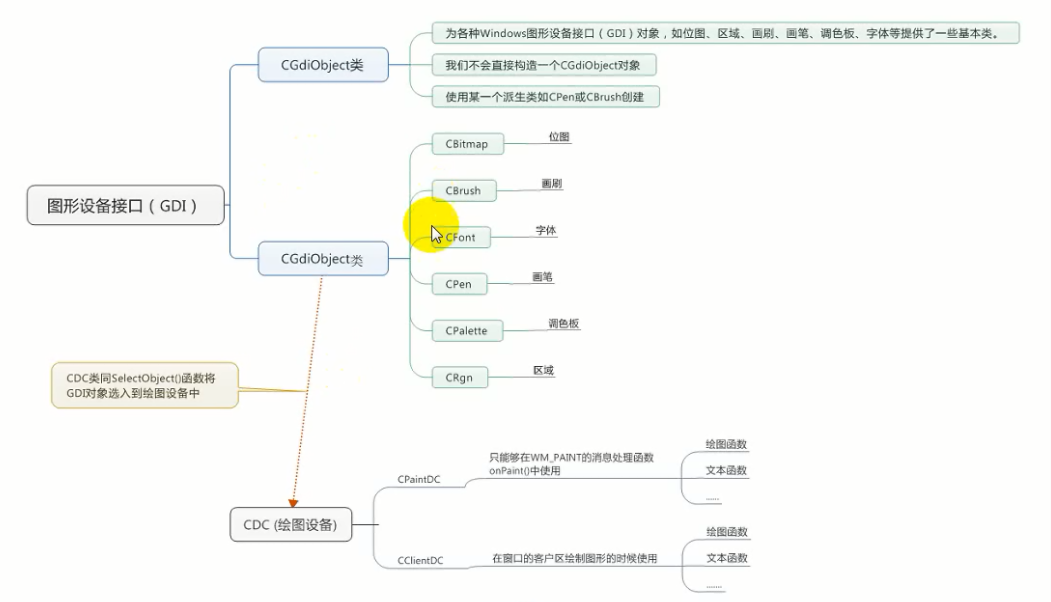``````void CMy02画图View::OnDraw(CDC* pDC)
{
CMy02画图Doc* pDoc = GetDocument();
ASSERT_VALID(pDoc);
if (!pDoc)
return;
//PS_DASH:虚线  只有当画笔宽度小于1或者更小的时候才有效
//10:线宽
CPen pen(PS_DASH,1,RGB(255,0,0));
//有返回值，回复原来的状态
pDC->SelectObject(&pen);

pDC->MoveTo(10, 10);
pDC->LineTo(120, 20);

//画圆形
pDC->Ellipse(20, 20, 120, 120);
//画刷 填充颜色
CBrush brush(RGB(255, 0, 0));
pDC->SelectObject(&brush);
pDC->Ellipse(20, 20, 120, 120);

//定义一个位图对象
CBitmap bitmap;
//加载位图
CBrush brush2(&bitmap);
pDC->SelectObject(&brush);
pDC->Ellipse(120, 20, 240, 240);
//设置字体
CFont font;
font.CreatePointFont(1000, TEXT("楷体"));
pDC->SelectObject(&font);
pDC->TextOutW(100, 100, TEXT("HELLO,MIKE"));
// TODO: 在此处为本机数据添加绘制代码
}

``````

``````//窗口创建后自动调用
int CMy03编辑View::OnCreate(LPCREATESTRUCT lpCreateStruct)
{
if (CView::OnCreate(lpCreateStruct) == -1)
return -1;

// TODO:  在此添加您专用的创建代码
//获取字体信息

CClientDC dc(this);

//字体信息结构体
TEXTMETRIC tm;
dc.GetTextMetrics(&tm);

//继承于CWnd
//创建插入符  要获取字体信息来进行插入符的操作
//第一个参数平均字符的宽度 字符高度
CreateSolidCaret(tm.tmAveCharWidth/8,tm.tmHeight);

//显示插入符
ShowCaret();

return 0;
}

void CMy03编辑View::OnLButtonDown(UINT nFlags, CPoint point)
{
// TODO: 在此添加消息处理程序代码和/或调用默认值

//设置鼠标点击的插入符位置
SetCaretPos(point);
//保存点击坐标
m_point = point;
//清空
m_str.Empty();

CView::OnLButtonDown(nFlags, point);
}

void CMy03编辑View::OnChar(UINT nChar, UINT nRepCnt, UINT nFlags)
{
// TODO: 在此添加消息处理程序代码和/或调用默认值
//点击键盘进行再插入符的地方进行输入字符串
//创建一个成员变量
CClientDC dc(this);

//换行  字符串为空，没有高度
if (nChar == VK_RETURN) {
m_str.Empty();
//获取字体信息
TEXTMETRIC tm;
dc.GetTextMetrics(&tm);
m_point.y = m_point.y + tm.tmHeight;
}
else if (nChar == VK_BACK) {
//获取背景色，用背景色来盖住
COLORREF color = dc.GetBkColor();
//设置写字颜色
COLORREF oldColor = dc.SetTextColor(color);
//白色重写一次
dc.TextOutW(m_point.x, m_point.y, m_str);
//去掉最后一个字符
m_str = m_str.Left(m_str.GetLength() - 1);
dc.SetTextColor(oldColor);

}
else {
m_str += (TCHAR)nChar;
}

//获取字符串的长度,插入符也跟着移动，起点不能变，所以用局部变量
CSize size = dc.GetTextExtent(m_str);
int x = m_point.x + size.cx;
int y = m_point.y;
SetCaretPos(CPoint(x, y));
dc.TextOutW(m_point.x, m_point.y, m_str);

CView::OnChar(nChar, nRepCnt, nFlags);
}

``````

``````// CMy04定时器View 消息处理程序

int CMy04定时器View::OnCreate(LPCREATESTRUCT lpCreateStruct)
{
if (CView::OnCreate(lpCreateStruct) == -1)
return -1;

// TODO:  在此添加您专用的创建代码

//设置定时器 第一个参数定时器ID 时间间隔以毫秒为单位
//第三个回调函数一般不用  NULL代表系统默认的处理函数,每隔100毫米，触发WM_TIMER
SetTimer(1,100,NULL);
return 0;
}

void CMy04定时器View::OnTimer(UINT_PTR nIDEvent)
{
// TODO: 在此添加消息处理程序代码和/或调用默认值
if (nIDEvent = 1) {
#if 0
static int i = 0;
i++;
CString str;
str.Format(TEXT("%d"), i);
if (i == 5) {
KillTimer(1);
}
MessageBox(str);
#endif
static int w = 0;
w += 5;
CString str = TEXT("塑造自己的过程很疼，但是你可以得到一个更好的自己");
CClientDC dc(this);
//获取字符串的长度(宽度)
CSize size = dc.GetTextExtent(str);
//如果宽度大于字符串的宽度，重新开始
if (w > size.cx) {
w = 0;
//重新刷新窗口
Invalidate();
}
//覆盖的矩形范围
int x = 30;
int y = 30;
CRect rect(x, y, x + w, y+size.cy);
//黑色写一次
dc.TextOutW(x, y, str);
//设置字体颜色
dc.SetTextColor(RGB(255, 0, 0));
dc.DrawText(str, &rect, DT_LEFT);
}
CView::OnTimer(nIDEvent);
}

``````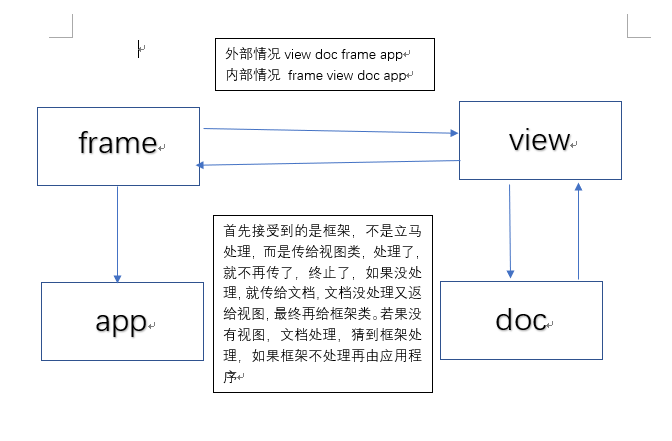CWnd可以接受任何消息
CCmdTarget不能接受标准消息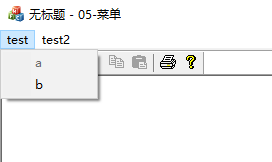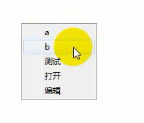``````//标志
//获取菜单，先获取菜单栏，再获取子菜单，相当于数组下标
//标志"新建"可以通过位置，也可以通过ID
//第一个参数由位置来决定
//第一个参数由ID来决定后面是打钩
//设置默认项 加粗 一个菜单上只有一个默认的
//第二个参数是FALSE代表ID 否则代表位置
//分隔符也算一个菜单的
//移除菜单项
//加载菜单
//分离  保证不释放
return 0;

//a的更新，菜单更新内部自动更新
void CMainFrame::OnUpdateTestA(CCmdUI *pCmdUI)
{
// TODO: 在此添加命令更新用户界面处理程序代码
if (true == m_isUpdate) {
pCmdUI->Enable(TRUE);
}
else {
pCmdUI->Enable(FALSE);
}
}

//b的处理函数
void CMainFrame::OnTestB()
{
// TODO: 在此添加命令处理程序代码
//bool类型
m_isUpdate = !m_isUpdate;
}

``````
``````//视图鼠标左键按下
void CMy05菜单View::OnLButtonDown(UINT nFlags, CPoint point)
{
// TODO: 在此添加消息处理程序代码和/或调用默认值
//在视图当中进行得创建菜单1.获取主菜单2.获取子菜单
//子菜单作为快捷菜单，右击菜单,坐标应该相对于屏幕
//坐标转换
ClientToScreen(&point);

CView::OnLButtonDown(nFlags, point);
}

``````

``````CMainFrame::CMainFrame()
{
//获取应用程序加载图标,AfxGetApp()获取应用程序
// TODO: 在此添加成员初始化代码
}

//在框架类里面的ONCREATE()里面设置定时器，然后在消息中添加回调函数OnTimer()
SetTimer(1, 500, NULL);

void CMainFrame::OnTimer(UINT_PTR nIDEvent)
{
// TODO: 在此添加消息处理程序代码和/或调用默认值

static int i = 0;
//改变标题图标
SetClassLong(m_hWnd, GCL_HICON, (LONG)icon[i]);
i++;
if (i == 4) {
i = 0;
}
CFrameWnd::OnTimer(nIDEvent);
}

``````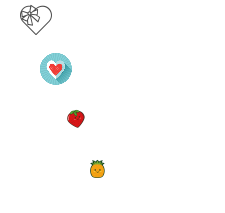``````	//OnCreate()函数里面
SetTimer(1, 500, NULL);

//加载图标  不知道绘图和这个函数谁先调用,所以放在构造函数里面
x1 = x2 = x3 = x4 = 30;
y1 = 30;

void CMy07作业View::OnTimer(UINT_PTR nIDEvent)
{
// TODO: 在此添加消息处理程序代码和/或调用默认值
if (1 == nIDEvent) {
x1 += 20;
x2 += 40;
x3 += 60;
x4 += 80;
//越界判断，获取客户区范围
CRect rect;
GetClientRect(&rect);
if (x1 > rect.Width()) {
x1 = 30;
}
if (x2 > rect.Width()) {
x2 = 30;
}
if (x3 > rect.Width()) {
x3 = 30;
}
if (x4 > rect.Width()) {
x4 = 30;
}
//刷新
Invalidate();
}
CView::OnTimer(nIDEvent);
}

void CMy07作业View::On32771()
{
// TODO: 在此添加命令处理程序代码
x1 = 30;
x2 = 30;
x3 = 30;
x4 = 30;
//刷新窗口
Invalidate();
}

``````

### Tomcat指定JDK版本_小志的博客的博客-程序员宅基地_tomcat设置jdk版本

windows1、找到bin下的setclasspath.bat文件；在文件的开始出添加如下代码：set JAVA_HOME=D:\Java\jdk7\jdk1.7.0_51set JRE_HOME=D:\Java\jdk7\jre7 linux2、在bin下找到setclasspath.sh，在文件的最开始加入如下代码：set JAVA_HOME=/opt/jdk/Ja...

### 简单易懂的事务四大特性ACID_Step_Top的博客-程序员宅基地

MySQL 事务事务简介 事务Transaction，是指作为一个基本工作单元执行的一系列SQL语句的操作，要么完全地执行，要么完全地都不执行。从一个帐号扣款并使另一个帐号增款，这两个操作要么都执行，要么都不执行。这两个操作必须保证全部成功或者什么都不做，不允许出现成功1个失败一个的情况。事务四大特性ACID原子性(Atomicity)一致性(Consistenc...

### Bugku-WEB篇(50')_东方与君语的博客-程序员宅基地

Bugku-WEB篇50分域名解析分析步骤域名解析分析计算机首先拿到域名后是先去本机的hosts文件（不知道的自行百度）找有没有对应的ip，没有再去访问DNShosts文件是一个没有扩展名的系统文件，它的主要作用是能加快域名解析，还可以屏蔽网站等。步骤在hosts添加上 123.206.87.240 flag.baidu.com（位置：c:\windows\system32\dri...

### The difference between d8&d9's constants def in asm shaders_一麒麟子一的博客-程序员宅基地

The difference between d8&amp;d9's constants def in asm shaders :::::Pick from dx9 sdkThe behavior of shader constants has changed between Direct3D 8 and Direct3D 9.F...